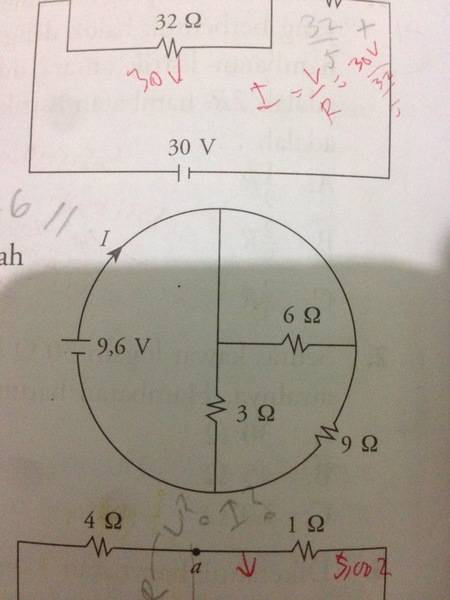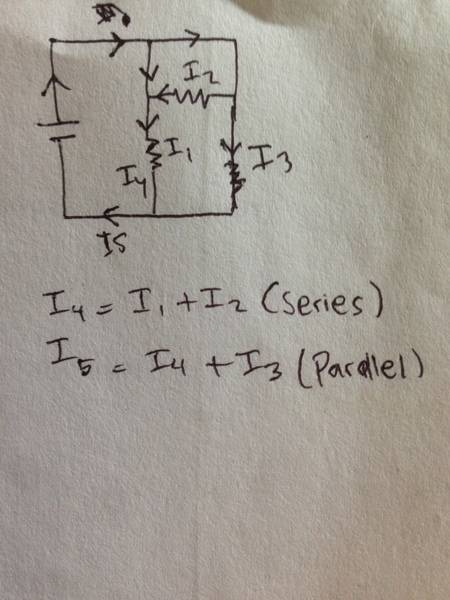# Find the current I in this electrical circuit

## Homework StatementV = I.R

## The Attempt at a Solution

6 ohm and 3 ohm are series = b
Then b pararel to 9ohm = c = total resistance
b = 9ohm
c = 9/2 ohm
I = V/R
I = 9.6/c
I = 2,13 A

Can you help me?
Is it right? My friend's answer is 0,8

#### Attachments

BvU
Homework Helper
If you leave out the 3 and 6 the current is already more than 1 A, so your friend must be wrong•Helly123
If you leave out the 3 and 6 the current is already more than 1 A, so your friend must be wrongBvU
Homework Helper
I'm not a betting man, nor a teacher. You have to convince yourself !

BvU
Homework Helper
Le me ask: what do you get when you leave out the 6 and the 9 ?Le me ask: what do you get when you leave out the 6 and the 9 ?I = V/R
I = 9.6/9 = 1.06 ??

BvU
Homework Helper
What about the wire from the top to the 3 ohm ? Wouldn't the current prefer to through that instead of through the 6 ohm ?
( leaving out the 6 and 9 leaves only the 3 ! )

cnh1995
Homework Helper
Gold Member
6 ohm and 3 ohm are series = b
They are not.

Can you see anything special about the 6 ohm resistor?

•Asymptotic
What about the wire from the top to the 3 ohm ? Wouldn't the current prefer to through that instead of through the 6 ohm ?
( leaving out the 6 and 9 leaves only the 3 ! )
Wouldn't the current go to both branches? Im still really not good at it. But why only 3?
I = 9.6/3 = 3.2 A?

They are not.

Can you see anything special about the 6 ohm resistor?
Yes, i think so. Maybe because the current from 1st branch go to 6ohm, and the current from 2nd branch go to 6 ohm too? So they're not series...?
If pararel then I = 9.6 / (18/11) = 5.8 A?

stockzahn
Homework Helper
Gold Member
What normally works for me is to re-draw the circuit (e.g. in the drawing I attached). Maybe now you can see what cnh1995 meant with "special" on the 6Ω-resistance?

#### Attachments

•Helly123 and Asymptotic
BvU
Homework Helper
Yes, i think so. Maybe because the current from 1st branch go to 6ohm, and the current from 2nd branch go to 6 ohm too? So they're not series...?
If pararel then I = 9.6 / (18/11) = 5.8 A?
The word is parallel.
Hwat is 11/18 ?
How far are you now ? Can you see the 6 ohm is short-circuited ? There is a wire from one end to the other. That wire makes the potential at both ends equal -- no current through the resistor. You can leave it out of the diagram without anything changeing. Then things look simple again ...

•Helly123
The word is parallel.
Hwat is 11/18 ?
How far are you now ? Can you see the 6 ohm is short-circuited ? There is a wire from one end to the other. That wire makes the potential at both ends equal -- no current through the resistor. You can leave it out of the diagram without anything changeing. Then things look simple again ...
Oh parallel.. thanks
3ohm and 9ohm are parallel, the total resistance is 9/4
I = 9.6 / (9/4)
I = 4.2 A is it?

BvU
Homework Helper
As in #4: you have to convince yourself !
(But I like this one a lot!)

As in #4: you have to convince yourself !
(But I like this one a lot!)
Ok.. we can conclude it as 4.2 A. Thank you

•BvU
As in #4: you have to convince yourself !
(But I like this one a lot!)
To be honest, i think i still get wrong. My answer is not among the options..

gneill
Mentor
To be honest, i think i still get wrong. My answer is not among the options..
What options are available?

cnh1995
Homework Helper
Gold Member
To be honest, i think i still get wrong. My answer is not among the options..
I = 9.6 / (9/4)
I = 4.2 A is it?
You have chopped off some decimal places.
What are the options?

epenguin
Homework Helper
Gold Member
Is an option 5⅓ A?

mpresic2
What is the potential difference across the six ohm resistor. Under what circumstances can you remove the six ohm resistor from the circuit

BvU
Homework Helper
Wouldn't the current go to both branches? Im still really not good at it. But why only 3?
I = 9.6/3 = 3.2 A?
So 3.2 A for the branch with the 3 ##\Omega## only.
Ok.. we can conclude it as 4.2 A. Thank you
That would be the 3.2 we had already plus 1 A for the 9 ##\Omega## resistor ? But how much is 9.6 V / 9 ##\Omega## ?

•Helly123
Options are 1/5A, 4/5A, 1/3A, 1A, 2A
I think thatR series = 6 + 3 = 9ohm (I3)
R parallel = 1/9 + 1/9 = 2/9 ohm
R total = 9/2 ohm (I5)
I = V/R = 9.6 / (4.5) = 2.1A
Close to options 2A ? But im not sure

#### Attachments

BvU
Homework Helper
Options are simply wrong. But from a number of posts in this threadyou can conclude that teacher isn't the only one in errorPerhaps you can see for yourself if you first erase the I and then add some V in your picture: both ends of R2 (the 6 ##\Omega) see the same V so I2 = 0 !
And then your I5 remains standing as correct and easy to calculate !

•Helly123
epenguin
Homework Helper
Gold Member
I think I gave the right answer in #19, but anyway you are nearly there now. It is certainly unhelpful when books or teachers give wrong answers and the student is banging his head trying to find the wrong answer! It is not the only example of that I have come across here recently.

I don’t know whether this is the only reason, anyway this calculation is taking far too long! I think we have got that the 6Ω resistor has no potential difference across it, there is no current in it, it might as well not be there. So all you have we have is a single voltage source and two resistors in parallel. But that is still being found, and is often found by students, unnecessarily difficult. Let me just give my take and tips on why is simple resistive (and capacitive) steady current circuits are found more difficult than they need be.

They are found unnecessarily difficult, in my guess, because students are taught something about reciprocal resistances being the sum of other reciprocal resistances or something, which sounds arcane, mathematical, and calculational, distanced from the simple physics. Which is that current comes out of one pole of the source, divides into streams, which then reunite and the same current returns to the other pole of the source.The sum of the currents in the different streams add up to the total current at the sources. (In the context, an almost self evident principle dignified by the name Kirchoff’s Current Law or or Conservation of Charge.)

You can just calculate each of the two currents in this problem separately. Then add them together for the total current.

Didacts have maybe tried to make this simpler by a formula, but I’d say experience shows they have made it more difficult for students.

When you have got used to adding up currents in parallel parts, which won’t take long, you may realise that parallel branches with the same potential difference across them are acting like a single conductor where the ability to conduct a current – the conductance - is the sum of the conductance in each branch. Conductance, G, of a conductor is defined as
I = GV
(You can call this a definition. Many people would call that equation Ohm’s Law. Really what Ohm’s law - a generalisation from experimental findings - says is that for conductors made of many materials, and over a wide range of conditions, G is a constant.)
Then the way currents in parallel add up mean that conductances in parallel add up. I.e. several conductors G1, G2,... with the same potential difference across them conduct in total just as much as a single conductor G, with effective conductance (aka ‘ equivalent conductance‘)
G = G1 + G2 +...
which hopefully has a self-evident quality about it.

Obviously from the equation above, conductance G is the reciprocal of resistance R. I guess dicacts have tried to not overburden students with too many terms and more or less eliminate ‘conductance’ from their terminology, but the term is suggestive so I think they have lost more than they gained.

When resistors are in series on the other hand, it is potential differences that add up, and the resistance concept is more useful as the total resistance of a series of resistors is the sum of resistances.

My other tips for student circuit analysis:

Have an eye for and use any symmetry in a circuit to simplify calculation. (I only got involved in homework help for circuits because I was seeing students making unnecessarily complicated calculations which could be simplified by symmetry.)

Use rational numbers like 5⅓ in preference to decimals. Using decimals will often be wiping out information. And decimals are boring. Rationals bear a trace of the original circuit problem. (These are my reasons - I won’t make exaggerated claims for accuracy. Just once in 250 times when making accurate calculation on complicated circuit you might gain an accuracy in the third significant figure by using rationals, and of course the input data is generally not that accurate, it’s just a point of principle.) Decimals are good only for the final answer, because measuring devices use decimals.

•Tom.G and Helly123
BvU
•